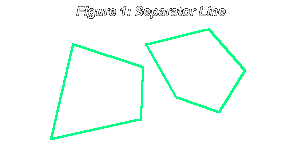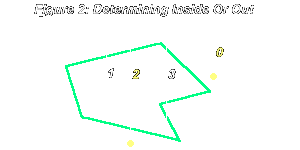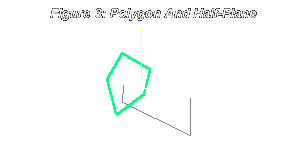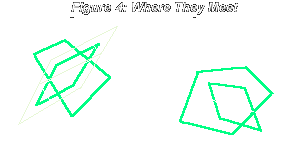Theory & Practice - Issue 02 - Collision Detection - Part 2 by (24 May 2000) Return to The Archives
 IntroductionThis time I talk about something I didn't cover in the last discussion, namely collisions with polygons. Most 3D engines nowadays are polygon-based, because building (hollow) objects from polygons and drawing them is relatively straightforward, and lends itself well to modeling. Modern 3D hardware can texturemap, transform and otherwise manipulate your polygons. But it's currently up to you to implement stuff like collision detection in your projects. So here I go, talking about polygon collisions.

 Polygons In 2DWe've talked about bounding boxes and we've talked about bounding circles. But often your objects are polygonal, these tests might be too inaccurate for final computation of collision between two objects. This is especially the case in 3D engines, where most objects are indeed made up of polygons. While bounding box and circle tests are good as preliminary "no/maybe" tests, you will later want to follow up the "maybe" by going through groups of polygons in an object and seeing whether the polygons do, in fact, intersect.So why are we doing polygon intersection in 2D? Because we should know how it works before going on to 3D, and because in some cases polygon collisions in 3D can reduce to collision in 2D, and therefore to being solved using these 2D techniques.In the circle, we took advantage of the fact that all the points are equidistant from the center. In the box, we saw that the distance between two opposing lines is constant, and used that. But suppose we had twoarbitrary n-gons; how can we find out whether they intersect?We are going to make our problem-solving lives easier by imposing one restriction: the polygons are convex. That means, if you drew a line between any two points in the polygon, no part of the line would be outside the polygon. Another way of saying that is: for every vertex in the polygon you can represent the polygon as a triangle fan with the vertex as the root.So we have two convex polygons. The idea is, if we can find a line that separates them, then they do not intersect. In other words, all of the vertices of polygon 1 are on one side of the line, and all of the vertices of polygon 2 are on the other. It just so happens that, if the polygons are convex, the line along an edge of one of the polygons will work. See the diagram below:So basically, here's what you do:For each vertex A in polygon 1, consider the line to the next vertex B. Let's say the slope of the line is (3, 5). Now, we know that all the vertices in polygon 1 are on one side of the line. We have to find out whether all the vertices in polygon 2 are on the other side of AB. To get the distance of a point to the line, we project it onto the line's perpendicular vector, which in this case can be either (3, -5) or (-3, 5). We first test the distance from the line to one vertex in polygon 1 (one which does not lie on the line). This is to see what the sign is, since we have to know what the "other side" sign is. Then we test the distance from AB to each vertex in polygon 2. If each vertex is on the other side of the line, then the line separates the two polygons, and therefore they don't intersect. If no such line was found, we do the same for each edge AB in polygon 2, since perhaps polygon 2 contains such a line separating both polygons.Notice the relationship between the number of vertices in the polygons and the amount of calculation in worst-case scenario. If we had one x-gon and one y-gon, then we'd have to check at most x+y edges, and for each of those edges check either x+1 vertices or y+1 vertices for distance. But overall it's not bad. Especially if you're doing this for two triangles, in which case there are 6 edges and 4 checks for each, for a total of 24 checks per pair of triangles.

 Point In PolygonThere are various ways of figuring out whether a 2D point is in a 2D polygon. Some ways work for convex polygons, and some work for concave ones as well. I am going to present here a way that works for either concave or convex polygons, and is also quite efficient, requiring less computation than other methods.It is based on a rather simple idea. A polygon is a closed shape, and like any closed shape, to get out from the inside you must pass the boundary. Now, suppose you took your point and drew a ray in an arbitrary direction from it. If the ray would intersect an odd number of edges in the polygon, then the point is inside the polygon. Otherwise it's outside.You can see why this is true: if the point is inside the polygon, as we travel along the ray, we get out, then get in, then get out, etc... since the polygon is finite in size we will eventually end up out of it, and then we would have crossed an odd number of edges. If the point is outside, our ray either crosses no boundaries at all (0 is an even number) or it would go in and then out, and of course it would eventually break away from the polygon completely.Putting this into an algorithm isn't very hard. The main idea here is to figure out, given a line, on which side of the line a vertex is located.Let's label everything so we know what we're talking about. We have a point A, which is being tested for whether it is inside the polygon. The polygon has vertices B0 through Bn-1. Now we take a ray that begins at A and goes off in an arbitrary direction, for example (A.x + 2t, A.y + 5t), where t >= 0. Call it C. The perpendicular vector to this is (5, -2) or (-5, 2), it doesn't matter which (they're negatives of each other). Let's call that vector D. Now we cycle through each vertex Bi in the polygon. We consider the vector from A to that vertex, which is (Bi.x-A.x, Bi.y-A.y), call it E. What we do for each vertex is take the dot product of D and E. For some vertices it might be positive, for others negative.Now that we have the dot product value for each vertex in the polygon, we look for pairs of consecutive vertices which are on different sides of the ray, i.e. the dot product values for one is negative, and the other positive. That is, for i = 0 to n-1, we consider the pairs Bi, B(i+1) mod n. If at the end the number of such pairs is odd, then the point was inside, otherwise it was outside.I want to make a comment: sometimes the arbitrary ray can actually pass exactly through a vertex! To handle that case, we actually have to test either whether one vertex < 0, the other >= 0 or one vertex <= 0, the other > 0, (but not one vertex <=0, the other >=0. You can easily see why.)Also, remember that we're dealing with a ray and not a line, so we also have to make sure we're only considering the edges that cross the ray. To do that we have to check the dot product of each vertex with the ray's vector, and if it's positive, we can proceed with that vertex. (We don't really care which way is positive, since we don't care which way the ray points). In the name of efficiency, this check should be done only on the vertex pairs that are found to have vertices on either side of the line. Once we've found such a pair, we make sure it's valid by also checking whether the edge really crosses the ray, and not the full line.

 Line In PolygonIf we were trying to see whether a portion of line segment was inside a polygon, we would proceed similarly. The "arbitrary" line C would be the segment being tested. Then its perpendicular vector D would be gotten, distances of vectors computed, etc. Then we'd cycle through the edges, and compute number of edges with vertices on different side of the line.After that, we'd pick an endpoint A of the line segment. For each vertex B in the polygon, we would check the value of AB dot C / |C|, which is the scalar projection of AB onto C. This is similar to how we checked for vertices being on one side of a ray. Except this time, we don't check whether the scalar projection result is positive, we check whether it is in the range 0 to |C| or -|C| to 0, depending on which endpoint we chose. To optimize things, we won't divide by |C| when checking the value of the scalar projection. Instead, we'll multiply the range by |C|, so it becomes 0 to |C|2 or -|C|2 to 0.Armed with this technique, we can actually return to the 2D polygon intersection problem, and solve it in a manner which is a little more efficient. Instead of checking each edge of each polygon and seeing whether it lies on a separating line, we can make the following test: cycle through all the edges in one of the polygons, and see if a part of the line segment which is the edge lies inside the other polygon. This way we only have to do 2xy checks in the worst case, given an x-gon and a y-gon. This is better than the x(y+1) + y(x+1) checks we would have to do otherwise, but not by much. And of course we stop whenever we find an edge that has a part located inside the other polygon.

 3D Point In PolygonNow, let's generalize these concepts into 3D. How do we find out whether a 3D point is on the surface of a planar polygon oriented somehow in 3D space? All the points, instead of having 2 coordinates, now have 3. Also, the arbitrary ray is now an arbitrary half-plane.

First we have to see whether the point is on the plane of the polygon. The plane equation is ax+by+cz+d = 0. Just plug the coordinates of the point into x, y and z in the equation and if we get 0 on the right side then it's on the plane. Otherwise the point is not on the surface of the polygon.

Next, how do we find an arbitrary plane that passes through a point (x1, y1, z1)? Well, remember the plane equation, ax+by+cz+d = 0. Take an arbitrary a, b and c. Then solve for d in the equation d = -(ax1 + by1 + cz1). That's it! [We have our arbitrary plane, and the polygon plane, and they intersect somewhere in a line.] Now we cycle through all the pairs of vertices as before, and find their distance from the plane. And if they're on different sides of the plane, we count that pair. If in the end, the number of these pairs is odd, the point is inside the polygon.One important equation in 3D rendering deals with the distance of a point to a plane. The distance of a point (x1, y1, z1) to a plane (ax+by+cz+d=0) is defined as the distance from that point to the closest point on that plane. The result is:

(1) Distance = ax1+by1+cz1+d

This equation is obtained from scalar-projecting a line -- from the point to an arbitrary point on the plane -- onto the normal vector of the plane. That big operation happens to reduce to equation 3. It's easy to remember, since you just have to substitute the point's coordinates into the plane equation's x, y and z. That is, of course, if the plane's normal vector is a unit vector (of length 1). Otherwise you also have to divide the expression by the length of the normal vector.

One thing to remember is, don't pick a plane that's exactly parallel to the plane of the polygon, since these planes will never intersect in a line, and all the vertices will be on one side of the plane. To ensure this doesn't happen, pick a, b and c for the arbitrary plane's equation based on the polygon plane's equation. For example, copy over the a and b, but add one to c. Also remember to normalize the vector of the plane you've created (make length 1), so you can do distance checking!

Also, remember that we really want a half-plane instead of a plane (like a ray instead of a line). So once we've found a vertex pair we also test whether it's on the positive side of the plane perpendicular to our half-plane.

 Example 1
Let's look at a problem that requires finding out whether a point is inside a polygon. Suppose you're a camera that's flying around in a 3D world. At any given point you have some x, y and z coordinates. You obviously don't want to fly through walls. Now, you are currently at a point (x1, y1, z1) and want to move to point (x2, y2, z2). Suppose you've isolated a polygon you might potentially collide with (perhaps using bounding boxes and so on). You want to check whether, if you indeed moved in a smooth line from (x1, y1, z1) to (x2, y2, z2) you'd pass through the polygon.

 Solution
This problem is similar to the ones we did earlier in 2D, such as the one with a point passing through a circle on its way to somewhere else. What we do first is figure out whether the point will pass through the polygon's plane, and if so, find the position of the point when it will be passing through the plane.

To do this, we apply equation 1 to find the distances of (x1, y1, z1) and (x2, y2, z2) from the plane. Let's call the distances d1 and d2. If they are both positive or both negative, then the point will not go through the polygon on its way from (x1, y1, z1) to (x2, y2, z2), and we are done. Otherwise, get the ratio d1/(d1-d2); once you interpolate between (x1, y1, z1) and (x2, y2, z2) according to that ratio, you get the position of the camera when it is passing through the plane. Let's call that position (x3, y3, z3).

Now that we have that point, let's see if it's inside the polygon. Let's say the polygon's plane equation is a1x+b1y+c1z+9=0. We form our "arbitrary" plane as follows: we say a2=a1, b2=b1, c2=c1+1. We then normalize the vector (a2, b2, c2). Now we obtain d2 by solving for it in d = -(a2x3 + b2y3 + c2z3). Now we have the half-plane we need, defined by a2, b2, c2 and d2.

Now we cycle through each vertex in the polygon, and store its distance from the half-plane. Having done that, we cycle through each vertex pair and count the number of pairs that have vertices on different sides of the half-plane. If the number of these pairs is odd, then the point is inside the polygon, otherwise it's not.

Finally, let's put things in perspective. If the camera doesn't cross the polygon's plane as it travels from (x1, y1, z1) to (x2, y2, z2), then the camera doesn't collide with the polygon. If the camera does cross the polygon's plane, we obtain the point (x3, y3, z3). If that point is inside the polygon, this means that the camera will pass through the polygon on its way from (x1, y1, z1) to (x2, y2, z2). Otherwise it won't.

 Polygons In 3DUsually in 3D, what you really want is to find out whether two volumes intersect. What you have are two polygon or triangle meshes that describe the surface area of those volumes. Luckily, it's easy to see that if the surface areas of two objects intersect, so do the objects themselves.

So you have to see whether any polygon in the first mesh intersects with any polygon in the second mesh. That can be a lot of comparisons! To reduce some of this load, only the meshes which receive the "maybe" result from bounding box or sphere collision tests are tested. Also, meshes may be subdivided and only the relevant parts of the meshes considered.

Anyway, it comes down to determining whether two polygons, arbitrarily oriented in 3D space, intersect or not. Let's say the polygons are planar, which means all the vertices in a given polygon lie on the same plane. Here's some pseudocode detailing what that involves:

 ```If the polygons are on the same plane Reduce to 2D polygon intersection tests Else { If the planes the polygons lie on intersect (in a line) { Check the span of polygon 1 along the line of intersection Check the span of polygon 2 along the line of intersection If the two spans intersect (1D intersection test) then the polygons intersect Otherwise, they don't } Else // planes are parallel The planes don't intersect, so neither do the polygons } ```

Here's a diagram showing when the polygons intersect in 3D:What we're really doing is reducing the space where the polygons can possibly meet. If they are both on the same plane, then we have two dimensions to check in. If they are on different planes that intersect, then we have one dimension to check in. The latter case is the easiest. We just check the spans of the polygons along the line of intersection. The former case is almost analogous to polygons in 2D, except we try to find a plane of separation rather than a line of separation. That's also not hard; since the perpendicular to that plane, the normal vector, is obtained by getting the cross product between the line connecting vertices A, B and the normal vector of the plane on which both polygons lie.

 ConclusionThis time we covered some common collision detection problems with polygons. Those of you making 3D engines might find this information helpful. In any case, let me know what you thought about this issue, and stay tuned! :-)

 Links And AcknowledgementsKurt Miller wrote a tutorial on basic collision detection with polygons, here on flipCode.Here's an article by Jeff Lander, that talks about different intersection tests for polygons.AdvancedCollision.com is actually a body shop that treats your car after a collision, hehehe.
 Article Series:Theory & Practice - Issue 00 - IntroductionTheory & Practice - Issue 01 - Collision DetectionTheory & Practice - Issue 02 - Collision Detection - Part 2Theory & Practice - Issue 03 - Curved SurfacesTheory & Practice - Issue 04 - Curved Surfaces, Part IITheory & Practice - Issue 05 - LandscapesTheory & Practice - Issue 06 - Event Handling Model